# Samacheer Kalvi Books: Tamilnadu State Board Text Books Solutions

## Samacheer Kalvi 9th Maths Chapter 2 Real Numbers Notes PDF Download: Tamil Nadu STD 9th Maths Chapter 2 Real Numbers NotesSamacheer Kalvi 9th Maths Chapter 2 Real Numbers Notes PDF Download: Tamil Nadu STD 9th Maths Chapter 2 Real Numbers Notes

## Samacheer Kalvi 9th Maths Chapter 2 Real Numbers Notes PDF Download

We bring to you specially curated Samacheer Kalvi 9th Maths Chapter 2 Real Numbers Notes PDF which have been prepared by our subject experts after carefully following the trend of the exam in the last few years. The notes will not only serve for revision purposes, but also will have several cuts and easy methods to go about a difficult problem.

 Board Tamilnadu Board Study Material Notes Class Samacheer Kalvi 9th Maths Subject 9th Maths Chapter Chapter 2 Real Numbers Format PDF Provider Samacheer Kalvi Books

## How to Download Samacheer Kalvi 9th Maths Chapter 2 Real Numbers Notes PDFs?

2. Click on the Samacheer Kalvi 9th Maths Notes PDF.
3. Look for your preferred subject.
4. Now download the Samacheer Kalvi 9th Maths Chapter 2 Real Numbers notes PDF.

## Tamilnadu Samacheer Kalvi 9th Maths Solutions Chapter 2 Real Numbers Additional Questions

Exercise 2.1

Question 1.
Find only two rational numbers between 14 and 34.
Solution:
A rational number between 14 and 34 = 12 ( 14 + 34) = 12 (1) = 12
Another rational number between 12 and 34 = 12 ( 12 + 34) = 12 ( 2+34 = 312 × 54) = 58
The rational numbers 12 and 58 lies between 14 and 32 .

Question 2.
Solution:
Yes, since 02 = 0, (i.e) it can be written in the form pq where q ≠ 0

Exercise 2.2

Question 1.
Express the following decimal expansion is the form pq , where p and q are integers and q ≠ 0.
(i) 0.75
(ii) 0.625
(iii) 0.5625
(iv) 0.28
Solution: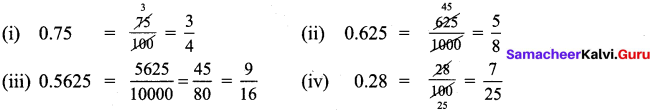Question 2.
Convert 0.9¯¯¯¯¯¯ to a rational number.
Solution:
(i) Let x = 0.9¯¯¯. Then x = 0.99999….
Multiplying by 10 on both sides, we get
10x = 9.99999….. = 9 + 0.9999….. = 9 + x
9x = 9
x = 1. That is, 0.9¯¯¯ = 1 (∵ 1 is rational number).

Exercise 2.3

Question 1.
Classify the following number as rational or irrational.
(i) 11−−√
(ii) 81−−√
(iii) 0.0625
(iv) 0.83¯¯¯
Solution:
(i) 11−−√ is an irrational number. (11 is not a perfect square number)
(ii) 81−−√ = 9 = 91 , a rational number.
(iii) 0.0625 is a terminating decimal
∴ 0. 0625 is a rational number.
(iv) 0.83¯¯¯ = 0.8333
The decimal expansion is non-terminating and recurring.
∴ 0.83¯¯¯ is a rational number.

Question 2.
Find the decimal expansion of 3–√.
Solution: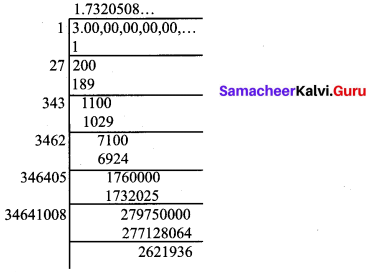Question 3.
Find any 4 irrational numbers between 14 and 13.
Solution:
14 = 0.25 and 13 = 0.3333 = 0.3¯¯¯
In between 0.25 and 0.3¯¯¯ there are infinitely many irrational numbers .
Four irrational numbers between 0.25 and 0.3¯¯¯ are
0.2601001000100001 ……
0.2701001000100001 ……
0.2801001000100001 …..
0.3101001000100001 ……

Exercise 2.4

Question 1.
Visualise 6.73¯¯¯ on the number line, upto 4 decimal places.
Solution:
We locate 6.73 on the number line, by the process of successive magnification.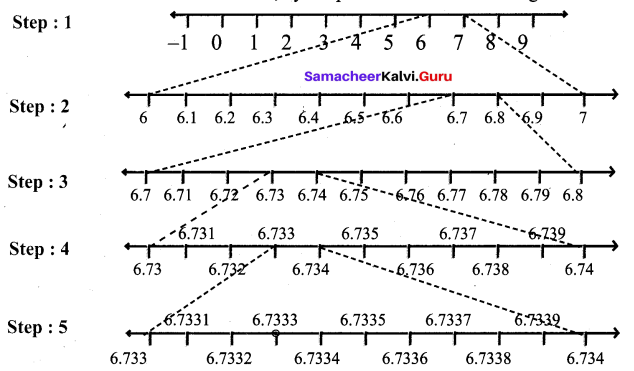Step 1 : First we note that 6.73¯¯¯ lies between 6 and 7.
Step 2 : Divide the portion between 6 and 7 into 10 equal parts and use a magnifying glass to visualise that 6.73¯¯¯ lies between 6.7 and 6.8.
Step 3 : Divide the portion between 6.7 and 6.8 into 10 equal parts and use a magnifying glass to visualise that 6.73¯¯¯ lies between 6.73 and 6.74.
Step 4 : Divide the portion between 6.73 and 6.74 into 10 equal parts and use a magnifying glass to visualise that 6.73¯¯¯ lies between 6.733 and 6.734.
Step 5 : Divide the portion between 6.733 and 6.734 into 10 equal parts and use a magnifying glass to visualise that 6.73¯¯¯ lies between 6.7332 and 6.7334.
We note that 6.73¯¯¯ is visualised closed to 6.7332 than to 6.7334.

Question 2.
Find whether x and y are rational or irrational in the following:
(i) a = 2 + 3–√, b = 2 – 3–√; x = a + b, y = a – b
(ii) a = 2–√ + 7, b = x = a + b, y = a – b
Solution:
(i) Given that a = 2 + 3–√, b = 2 – 3–√
x = a + b = (2+ 3–√) +(2 – 3–√) = 4, a rational number.
y = a – b = {2 + 3–√) – (2 – 3–√) = 23–√ , an irrational number.

(ii) Given that a = 2–√ + 7,b = 2–√ – 7
x = a + b = (2–√ + 7)+ (2–√ – 7) = 22–√, an irrational number.
y = a – b = (2–√ + 7 ) – (2–√ – 7) = 14, a rational number.

Exercise 2.5

Question 1.
Evaluate :
(i) 10-4
(ii) (19)-3
(iii) (0.01)-2
Solution: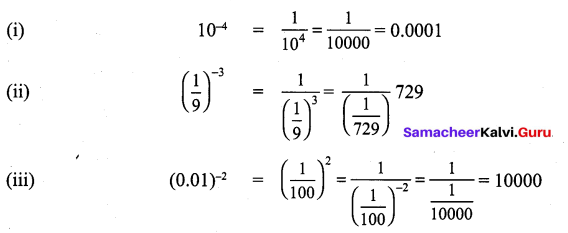Question 2.
Find the value of 62534 :
Solution: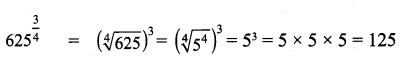Question 3.
Find the value of 729−56 :
Solution: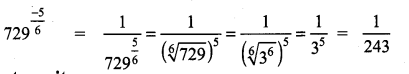Question 4.
Use a fractional index to write :
(i) (5125−−−√)7
(ii) 7–√3
Solution:
(i) (5125−−−√)7 = 12575
(ii) 7–√3 = 713

Exercise 2.6

Question 1.
Can you reduce the following numbers to surds of same order.
(i) 5–√
(ii) 5–√3
(iii) 5–√4
Solution: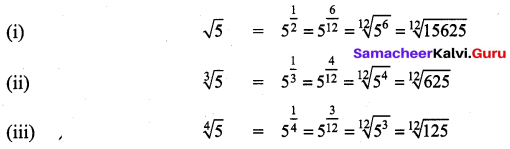Now the surds have same order

Question 2.
Express the following surds in its simplest form
(i) 27−−√
(ii) 128−−−√3
Solution: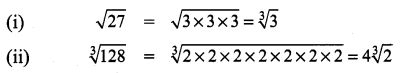Question 3.
Show that 2–√3 > 3–√5.
Solution: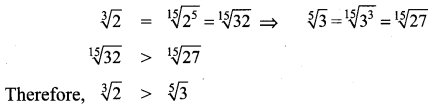Question 4.
Express the following surds in its simplest form 324−−−√4.
Solution: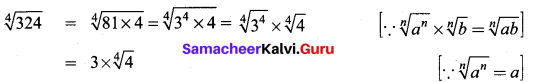order = 4 ; radicand = 4; Coefficient = 3

Question 5.
Simplify 63−−√ – 175−−−√ + 28−−√
Solution: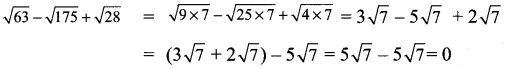Question 6.
Arrange in ascending order: 2–√3, 4–√2, 3–√4
Solution:
The order of the surds 2–√3, 4–√2, 3–√4 are 3, 2, 4
L.CM. of 3, 2, 4 = 12.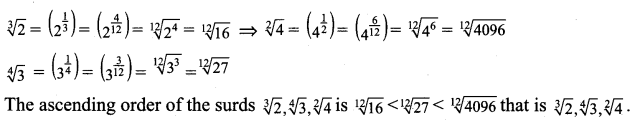Exercise 2.7

Question 1.
Subtract 67–√ from 97–√. Is the answer rational or irrational?
Solution:
97–√ – 67–√ = (9 – 6) 7–√ = 37–√

Question 2.
Simplify,: 44−−√ + 99−−√ – 275−−−√.
Solution: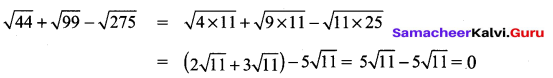Question 3.
Compute and give the answer in the simplest form : 3 162−−−√ x 7 50−−√ x 6 98−−√
Solution:
3 162−−−√ × 7 50−−√ × 6 98−−√ = (3×92–√×7×52–√×6×72–√)
= 3×7×6×9×5×7×2–√×2–√×2–√=793802–√

Exercise 2.8

Question 1.
Write in scientific notation : (60000000)3
Solution:
(60000000)3 = (6.0 × 107)4 = (6.0)4 × (107)4
= 1296 × 1028
= 1.296 × 103 × 1028 = 1.296 × 1031

Question 2.
Write in scientific notation : (0.00000004)3
Solution:
(0.00000004)3 = (4.0 × 10-8)3 = (4.0)3 × (10-8)3
= 64 × 10-24 = 6.4 × 10 × 10-24 = 6.4 × 10-23

Question 3.
Write in scientific notation : (500000)5 × (3000)3
Solution:
(500000)5 × (3000)3 = (5.0 × 105)3 × (3.0 × 103)3
= (5.0)2 × (105)× (3.0)3 × (103)3
= 25 × 1010 × 27 × 109 = 675 × 1019
= 675.0 × 1019 = 6.75 × 102 × 1019= 6.75 × 1021

Question 4.
Write in scientific notation : (6000000)3 ÷ (0.00003)2
Solution:
(6000000)3 + (0.00003)2 = (6.0 × 106)3 + (3.0 × 10-5)2
= (6.0 × 106)3 ÷ (3.0 × 10-5)2 = 216 × 1018 ÷ 9 × 10-10
= 216×1099×10−10
= 24 × 1018 × 1010 = 24 × 1028
= 24.0 × 1028 = 2.4 × 10 × 1028 = 2.4 × 1029

Exercise 2.9

Multiple Choice Questions :
Question 1.
A number having non-terminating and recurring decimal expansion is
(1) an integer
(2) a rational number
(3) an irrational number
(4) a whole number
Solution:
(2) a rational number
Hint:
Irrational number have nonterminating and non recurring decimal expansion.

Question 2.
If a number has a non-terminating and non-recurring decimal expansion, then it is
(1) a rational number
(2) a natural number
(3) an irrational number
(4) an integer
Solution:
(3) an irrational number
Hint: Rational number gave terminating or recurring and non-terminating decimal expansion.

Question 3.
Decimal form of −34 is
(1) -0.75
(2) -0.50
(3) -0.25
(4) -0.125
Solution:
(1) -0.75
Hint:
14 = 0.25; 12 = 0.5; 34 = 0.75

Question 4.
Which one of the following has a terminating decimal expansion?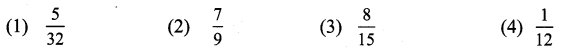Solution:
(1) 532
Hint:
32 = 25 ⇒ 532 has terminating decimal expansion

Question 5.
Which one of the following is an irrational number?
(1) π
(2) √9
(3) 14
(4) 15
Solution:
(1) π

Question 6.
Which one of the following are irrational numbers?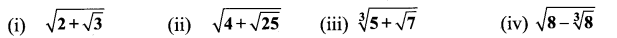(a) (ii), (iii) and (iv)
(b) (i), (ii) and (iv)
(c) (i), (ii) and (iii)
(d) (i), (iii) and (iv)
Solution:
(d) (i), (iii) and (iv)
Hint:
4+25−−√−−−−−−−√=9–√=3;8−8–√3−−−−−−√=8−2−−−−√=6–√

Question 7.
Which of the following is not an irrational number?
(1) 2–√
(2) 5–√
(3) 3–√
(4) 25−−√
Solution:
(4) 25−−√

Question 8.
In simple form, 54−−√3 is?
(1) 3 2–√3
(2) 3 27−−√3
(3) 3 2–√3
(4) 3–√
Solution:
(1) 3 2–√3

Question 9.
192−−−√3 + 24−−√3
(1) 36–√3
(2) 63–√3
(3) 3216−−−√3
(4) 3216−−−√6
Solution:
(2) 63–√3

Question 10.
521−−√ × 610−−√
(1) 30210−−−√
(2) 30
(3) 210−−−√
(4) 21030−−√
Solution:
(1) 30210−−−√

Text Book Activities

Activity – 1
Is it interesting to see this pattern? 4415−−−√=4415−−√ and 5524−−−√=5524−−√ Verify it. Can you frame 4 such new surds?
Solution: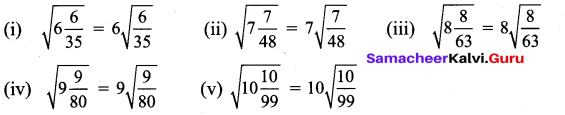Activity – 2
Take a graph sheet and mark O, A, B, C as follows: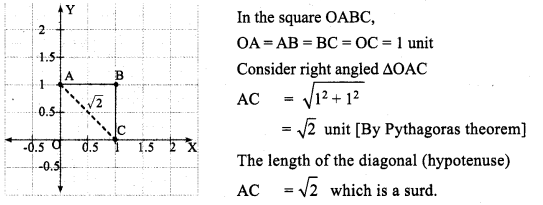Consider the following graphs: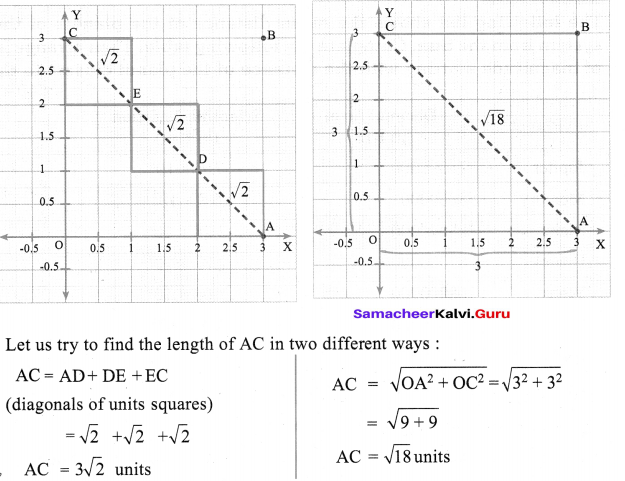Are they equal? Discuss. Can you verify the same by taking different squares of different lengths?
Solution: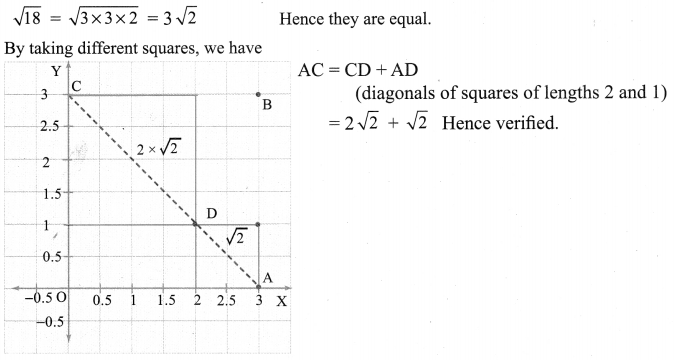Activity – 3
The following list shows the mean distance of the planets of the solar system from the Sun. Complete the following table. Then arrange in order of magnitude starting with the distance of the planet closest to the Sun.
Solution: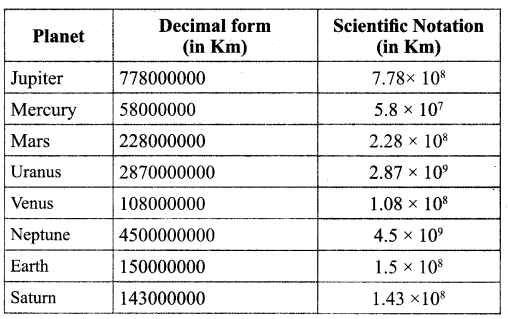Arrange the planets in order of distance from the sun.
Mercury, Venus, Earth, Mars, Jupiter, Saturn, Uranus, Neptune and Pluto.

## How to Prepare using Samacheer Kalvi 9th Maths Chapter 2 Real Numbers Notes PDF?

Students must prepare for the upcoming exams from Samacheer Kalvi 9th Maths Chapter 2 Real Numbers Notes PDF by following certain essential steps which are provided below.

• Use Samacheer Kalvi 9th Maths Chapter 2 Real Numbers notes by paying attention to facts and ideas.
• Pay attention to the important topics
• Refer TN Board books as well as the books recommended.
• Correctly follow the notes to reduce the number of questions being answered in the exam incorrectly
• Highlight and explain the concepts in details.

## Frequently Asked Questions on Samacheer Kalvi 9th Maths Chapter 2 Real Numbers Notes

#### How to use Samacheer Kalvi 9th Maths Chapter 2 Real Numbers Notes for preparation??

Read TN Board thoroughly, make separate notes for points you forget, formulae, reactions, diagrams. Highlight important points in the book itself and make use of the space provided in the margin to jot down other important points on the same topic from different sources.

#### How to make notes for Samacheer Kalvi 9th Maths Chapter 2 Real Numbers exam?

Read from hand-made notes prepared after understanding concepts, refrain from replicating from the textbook. Use highlighters for important points. Revise from these notes regularly and formulate your own tricks, shortcuts and mnemonics, mappings etc.
Share: